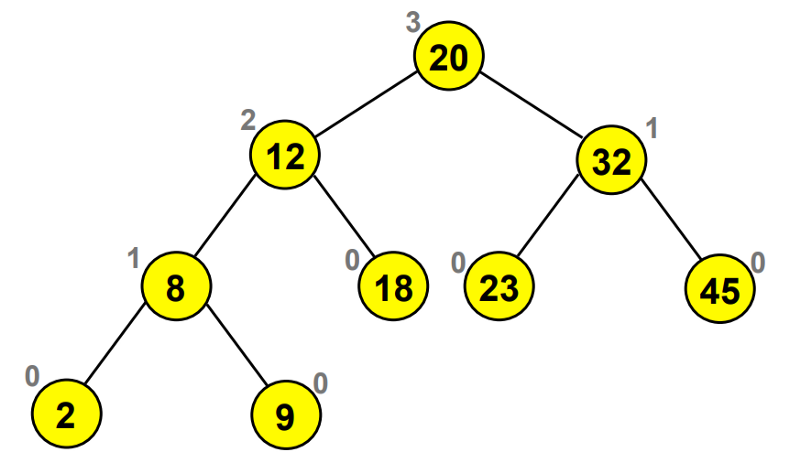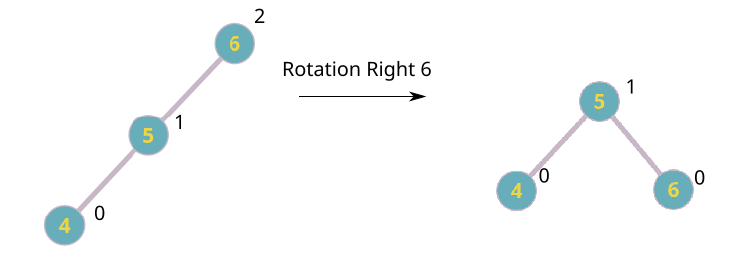by Tiago Antunes

# An introduction to trees in programming: the oxygen of efficient coding

Many times you wish to save information in your program and access it many times. And you’ll often store it in a very simple data structure: an array. And it often works really well! But sometimes it just takes a lot of time to finish.

And so, to optimize this kind of program, many smart people developed some weird things that we call data structures. Today I’ll be addressing some basics about this topic, and I’ll discuss one specific structure that is often asked about during coding interviews and makes everyone crazy: Trees.

I won’t be diving much into the code, only into the theory of how everything works. There are millions of code samples online, so just take a look at one after you understand how trees work!

### So what really is a data structure?

According to Wikipedia:

“ a data structure is a data organization and storage format that enables efficient access and modification”

This basically means that it’s nothing more than code written to create a complex way to store data. There are a lot of data structures that you can implement, and each one has a specific task. They can go from really simple ones — like linked lists — to really complex structures — like graphs.

Trees are complex enough to be really fast at what they do, but simple enough that that they’re understandable. And one thing they’re really good at is finding values with minimum memory usage.

### But how do you measure how efficient a data structure really is?

Have you ever seen some strange notation people use online like O(n)? That’s called Big O Notation, and it’s the standard way to evaluate the performance of structures and algorithms. The big O that we use is the representation of the worst-case scenario: having something that is O(n) (with n being the number of elements inside) means that in the worst case it takes time n, which is really good.

Inside the parenthesis, we wrote n which is the equivalent of writing the expression `y = x →` . It scales proportionally. But sometimes we have different expressions:

• O(1)
• O(log(n))
• O(n)
• O(n²)
• O(n³)
• O(n!)
• O(e^n)

The lower the result of a function, the more efficient a structure is.
There are multiple types of trees. We will be talking about BST (Binary-Search Trees) and AVL Trees (Auto balanced trees) which have different properties:As you can see AVL trees are much more efficient, but much more complex!

### Ok you talked about all this weird notation…so how do trees work?

The name tree comes from its real representation: it has a root, leaves and branches, and is often represented like this:Notice how 6 isn’t a leaf because it is not at the end of a branch!

There are a few denominations that we use, namely parent and child, which have a unique relationship. If x is parent of y then y is child of x. In this image, 2 is parent of 5, then 5 is child of 2. Each node — each position with a value — can only have 1 parent and 2 children.

But besides all this, there isn’t a pattern that is followed so this tree isn’t really that useful… So we should add a few more rules to make a good data structure.

#### Binary search trees

That’s when Binary-Search Trees come in! Instead of just randomly placing child nodes, they follow a specific order:

• If there are no nodes, then the first value that is entered becomes the root of the tree.
• If there are nodes, then the insertion takes the following steps: starting at the root, if the value you’re entering is smaller than the current node, go through the left branch, else go through the right one. If you’re in an empty place, then that’s where your value belongs!

This might feel a little confusing at the beginning, but let’s write some pseudo code to simplify it:

``//This code won't compile in any language (that I know of) and only serves to demonstrate how the code would look like``
``def insert(Node n, int v) {    if n is NULL:        return createNode(v)    else if n.value < v:        n.right = insert(n.right, v)    else:        n.left = insert(n.left, v)    return n}``

Now what’s happening here? First we check if the place where we are now is empty. If it is, we create a node in that place with the function `createNode`. If it’s not empty, then we must see where we should place our node.

This demo shows how it works:

This way we can search for any value in the tree without having to go through all the nodes. Great!

But it doesn’t always go as well as in the gif above. What if we get something like this?

In this case, the behavior of the tree makes you go through all of the nodes. That’s why a BST’s worst case efficiency is of O(n), which makes it slow.
Deleting from the BST is also easy:

• If a node has no children →remove the node
• If a node has one child →connect the parent node to its grandchild node and remove the node
• If a node has 2 children →substitute the node for its biggest child (the rightmost left child) → see image belowRemotion of number 12 happens by substitution of 12 by 9

So now you know everything you need about BST’s. Pretty cool huh?

### But what if you wanted to ALWAYS have an efficient tree? What would you do?

If you have that necessity, AVL trees can do that for you pretty well. In exchange, they are millions of times more complex than BST’s but follow the same organization as before.

An AVL tree is a self-balancing tree that has specific operations (called rotations) that allow the tree to stay balanced . This means that each node in the tree will have a difference of height between its two child branches of maximum 1.

With this, the tree will always have a height of log(n) (n being the number of elements) and this allows you to search for elements in a really efficient way.There are 11 elements and height is 4. So that means that the program would make at most 4 searches down! That’s really efficient as each level down hold the double the number of elements of its upper level

So now you see how good and perfect balanced trees are. But how to make them is the real question. I have mentioned the word depth before, so let’s first understand it.A leaf has height 0 and a non-leaf node has a height of the max of its children plus 1

Height is what allows us to understand if our tree is balanced or not. And with it we’re able to determine where the problem is and apply the balancing functions: rotations. These are really simple functions that consist of exchanging nodes between each other in order to remove the height difference, but they might look really strange at first.

There are 4 operations:

• Rotation Left
• Rotation Right
• Rotation Left-Right
• Rotation Right-LeftThe node where the children’s height was bigger than 1 suffers a rotation (imagine a blank place with a height of -1, if you calculate it: balance(6) = 1 - -1 = 2)

### Wow that was strange… how do rotations work?

Rotations are strange at first, and I suggest checking out some animations of them working.

Try with your own trees on this website: https://www.cs.usfca.edu/~galles/visualization/AVLtree.html

It allows you to dynamically see the rotations happening and is a great tool!
There are only four cases, so understanding them will be easy.